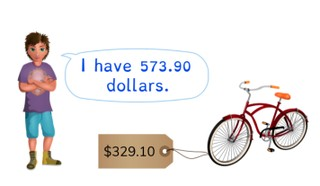Estimated and exact difference with decimal numbers with 1 or 2 decimal places

# Estimated and exact difference with decimal numbers with 1 or 2 decimal places8,000 schools use Gynzy92,000 teachers use Gynzy1,600,000 students use Gynzy

## General

Students learn to find the estimated and exact differences of decimal numbers with 1 or 2 decimal places.

5.NBT.B.7

## Relevance

It is important to be able to estimate the difference and find the exact difference of decimal numbers, so you can use the estimate to help check your exact difference. You can use this to help determine about how much money you should get back after buying something.

## Introduction

The interactive whiteboard shows a number line from 1 to 1 with a selection of decimal numbers. Ask students to determine where the decimal numbers belong on the number line. Draw a line from the decimal number to where it belongs on the number line. Make sure to remind students that a number with two decimal places is not always greater than a number with one decimal place.

## Development

To be able to estimate the difference with decimal numbers with 1 or 2 decimal places, students must be able to round decimal numbers to whole numbers. Explain that they can do this by looking on a number line to see which whole number a decimal number is closest to. You can also explain that with larger numbers it can be easier to round to a tens or hundreds number, to make the subtraction problem easier. Next explain how to subtract numbers with 1 decimal place. Show that you can do this on the number line when you split the subtrahend and take it away from the first number in parts. Show students that they can also subtract without a number line, by splitting both numbers and subtracting the whole numbers, subtracting the numbers after the decimal point, and then adding those differences together. Give students a problem in context and discuss how you can use both methods to find the difference. Repeat these steps and demonstrate to students how to subtract a decimal number with 2 decimal places.

To check that students are able to estimate the difference and find the exact difference of decimal numbers with 1 or 2 decimal places, you can ask the following questions.
- How do you round a number if you want to estimate a difference?
- How do you subtract decimal numbers if you want to know the exact difference?
- How can you check your exact difference?

## Guided practice

Students are given subtraction problems in which they are first asked to estimate the difference, and then asked to find the exact difference. They are first given subtraction problems with 1 decimal place, and then problems with 2 decimal places. They are also given story problems in which they are asked to estimate the difference as well as find the exact difference.

## Closing

Discuss with students that it is important to estimate the difference first because you can use that to check the difference you get when you solve for the exact difference, because the answers should be similar. Check that students are able to estimate the difference and find the exact difference using the given methods by giving students the subtraction problems and asking them to explain their strategy. Next three subtraction problems are given in which students are asked to first estimate, and then find the exact difference. You can erase the grey squares to reveal the answers. Ask students to explain their thinking.

## Teaching tips

Students who have difficulty with this learning goal can be supported by making use of a number line. They should first practice rounding decimal numbers to whole numbers, because then they build confidence that their estimated difference is a good check for their exact difference. You can also encourage students to take smaller jumps, or to split the subtrahend into smaller portions to take away from the minuend.

### The online teaching platform for interactive whiteboards and displays in schools

• Save time building lessons

• Manage the classroom more efficiently

• Increase student engagement